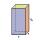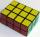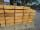# Stone

When Peter threw stone in a box of water he discovered that the water level has risen by 9 cm.

The box has a cuboid shape, the bottom has dimensions of 24 cm and 14 cm, height is 50 cm.

What volume has a stone?

Result

V =  3024 cm3

#### Solution:Leave us a comment of example and its solution (i.e. if it is still somewhat unclear...):Be the first to comment!#### To solve this example are needed these knowledge from mathematics:

Tip: Our volume units converter will help you with converion of volume units.

## Next similar examples:

1. Water poolWhat water level is in the pool shaped cuboid with bottom dimensions of 25 m and 10 meters, when is 3750hl water in the pool.
2. Fire tankHow deep is the fire tank with the dimensions of the bottom 7m and 12m, when filled with 420 m3 of water?
3. Concrete pillarHow many m³ of concrete is needed for the construction of the pillar shape of a regular tetrahedral prism, when a = 60 cm and the height of the pillar is 2 meters?
4. Reservoir 3How many cubic feet of water is stored in the reservoir that has a length of 200 feet, a width of 100 feet, an overflow depth of 32 feet, and a current water level of 24 feet?
5. Swimming pool 4The pool shaped cuboid measuring 12.5 m × 640 cm at the bottom is 960hl water. To what height in meters reaches the water level?
6. Water reservoirThe water tank has a cuboid with edges a= 1 m, b=2 m , c = 1 m. Calculate how many centimeters of water level falls, if we fill fifteen 12 liters cans.
7. AquariumAquarium is rectangular box with square base containing 76 liters of water. Length of base edge is 42 cm. To what height the water level goes?
8. Cuboid aquariumCuboid 25 times 30 cm. How long is third side if cuboid contains 30 liters of water?
9. HectolitersHow many hectoliters of water fits into cuboid tank with dimensions of a = 3.5 m b = 2.5 m c = 1.4 m?
10. Water lakeThe length of the lake water is 8 meters width 7 meters and depth 120 centimeters. How many liters of water can fit into the water lake?
11. Cuboid - simpleCalculate the surface area and volume of a cuboid if a = 8 cm, b = 14 cm and c = 6 cm.
12. Concrete boxThe concrete box with walls thick 5 cm has the following external dimensions: length 1.4 m, width 38 cm and height 42 cm. How many liters of soil can fit if I fill it to the brim?
13. Third dimensionCalculate the third dimension of the cuboid: a) V = 224 m3, a = 7 m, b = 4 m b) V = 216 dm3, a = 9 dm, c = 4 dm
14. Two cuboidsFind the volume of cuboidal box whose one edge is: a) 1.4m and b) 2.1dm
15. CuboidHow many times will increase the volume of a cuboid, if one dimension is twice larger, second dimension three times larger and third dimension four times lower?
16. Wood planks1 m3 of wood planks costs 179 EUR. How much will I pay for 11 planks measuring 6 cm, 10 cm and 6 m?
17. Rectangular prismIf i have a rectangular prism with a length of 1,000 cm, width of 30 cm and a height of 50 cm, what is the volume?